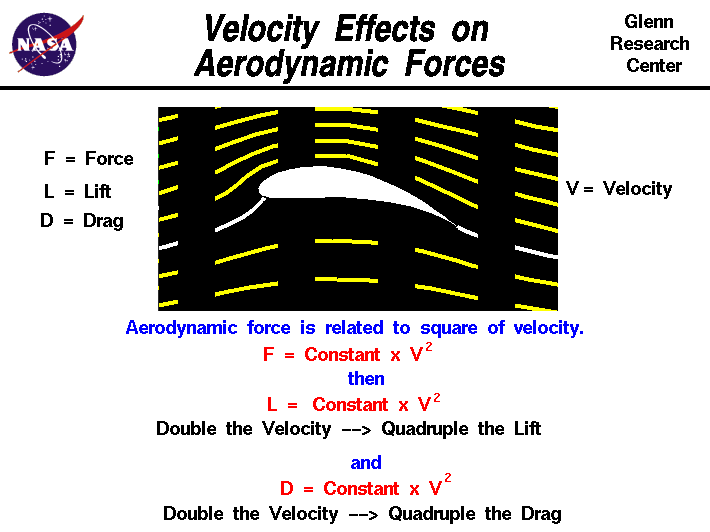+ Text Only Site
+ Non-Flash Version
+ Contact Glenn## Investigating Velocity Effects at Takeoff ProblemsIf so instructed by your teacher, print out a worksheet page for these problems.

I. For each of the tables below:

1. Determine the square of each velocity.
2. Enter the data in the table.
3. Start the FoilSim program.

a) Set the following conditions:

Airspeed = 100 mph
Altitude = 0 feet
Angle = 0 degrees
Thickness = 12.5%
Camber = 0
Area = 1.0 sq ft

b) Assume and enter the following for each aircraft:

Altitude = 0 feet
Angle = 0 degrees
Thickness = 12.5%
Camber = 5.0%

c) Enter the areas you determined for each aircraft (both wings) from the Wing Area Investigations Activity.
{Hint: Lift and Area are directly proportional. If the area is over 1000 sq. ft., then divide it by 10 and enter that number. When you get the lift, multiply it by 10 to get the real value!}

4. Record the lift generated by each entry in your table.5. Keeping in mind that the Lift is directly proportional to the square of the Velocity, calculate and record the Constant for each entry in each table.

 Wright Brothers Area of two wings: Velocity: Velocity Squared: Lift: Constant: 0 25 50 75 100 125 150 175 200 225 250

 F-18 Area of two wings: Velocity: Velocity Squared: Lift: Constant: 0 25 50 75 100 125 150 175 200 225 250

 (Your Aircraft) Area of two wings: Velocity: Velocity Squared: Lift: Constant: 0 25 50 75 100 125 150 175 200 225 250

6. Are the constants exactly the same for all entries in each individual table? Why or why not? List possible sources of error.
7. Are the constant values the same for each aircraft? Discuss and list some reasons for this.

II. Review the values entered in FoilSim.

1. Which values were kept the same for all aircraft in this activity?
2. Which values differed from aircraft to aircraft in this activity?
3. The "Constant" values you calculated come from the values that are held constant (not changed). Which values do you think are included in the "Constant" value for each aircraft?
4. Could these values ever be considered variables? If so, when and how? If not, why not?
5. Suppose your aircraft is loaded with cargo and extra fuel for a long flight so that it weighs 12500 pounds before takeoff. What airspeed is needed to produce enough lift to get your aircraft airborne? {Hint: On the ground the altitude and angle are both zero. Also, use the same camber and thickness as before since it's the same plane.}

Related Pages:
Standards
Worksheet
Lesson Index
Aerodynamics Index+ Inspector General Hotline + Equal Employment Opportunity Data Posted Pursuant to the No Fear Act + Budgets, Strategic Plans and Accountability Reports + Freedom of Information Act + The President's Management Agenda + NASA Privacy Statement, Disclaimer, and Accessibility CertificationEditor: Tom Benson NASA Official: Tom Benson Last Updated: Thu, Jun 12 04:47:15 PM EDT 2014 + Contact Glenn